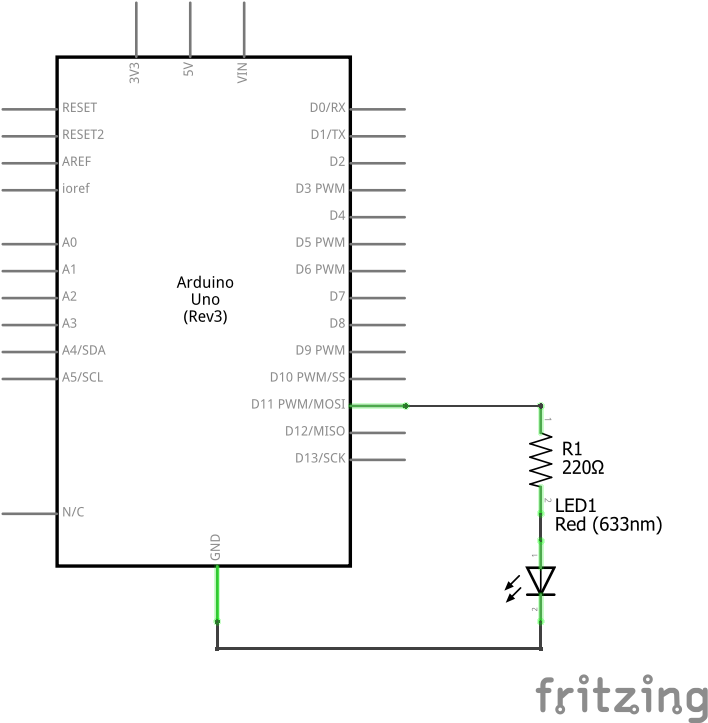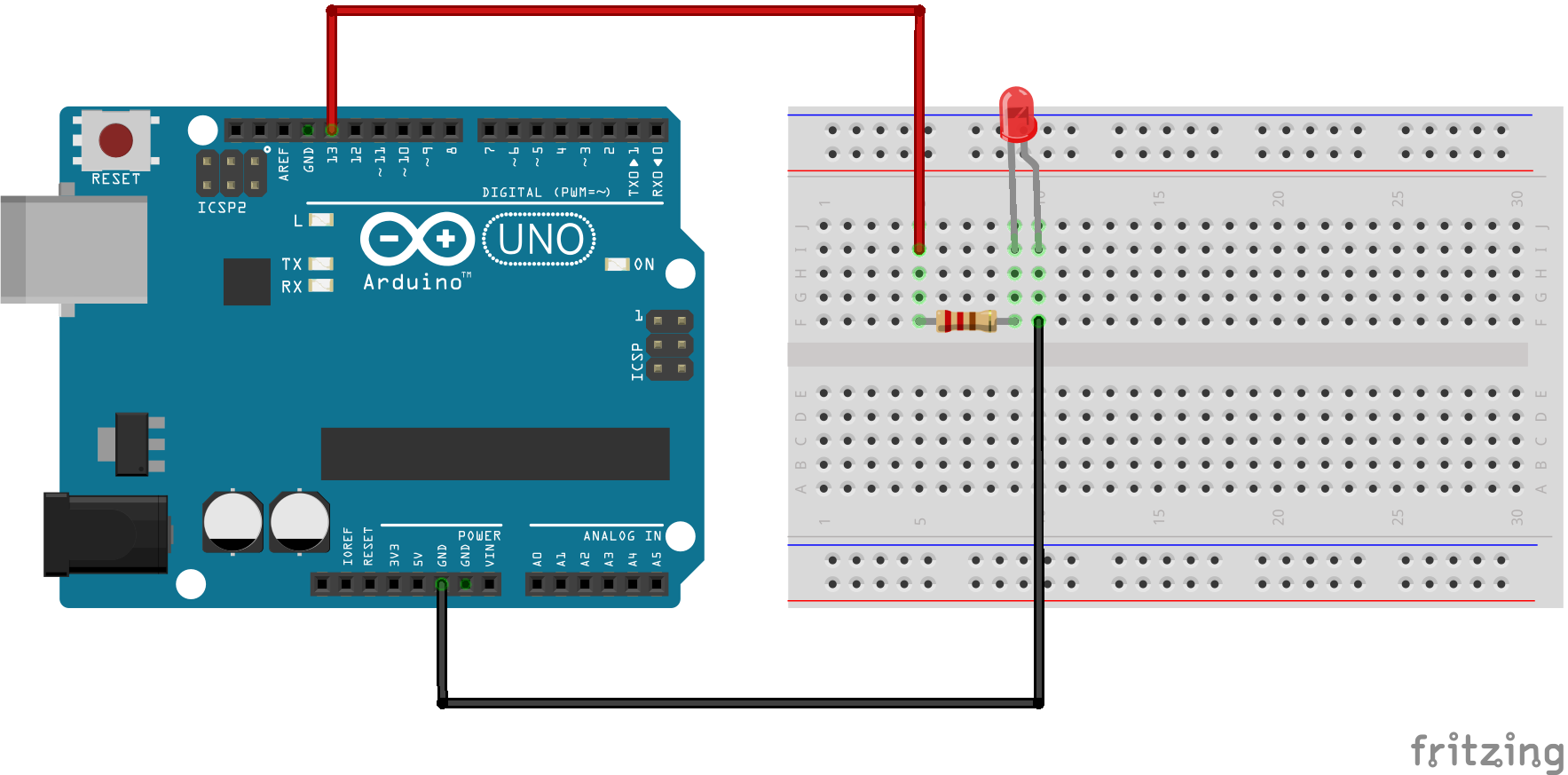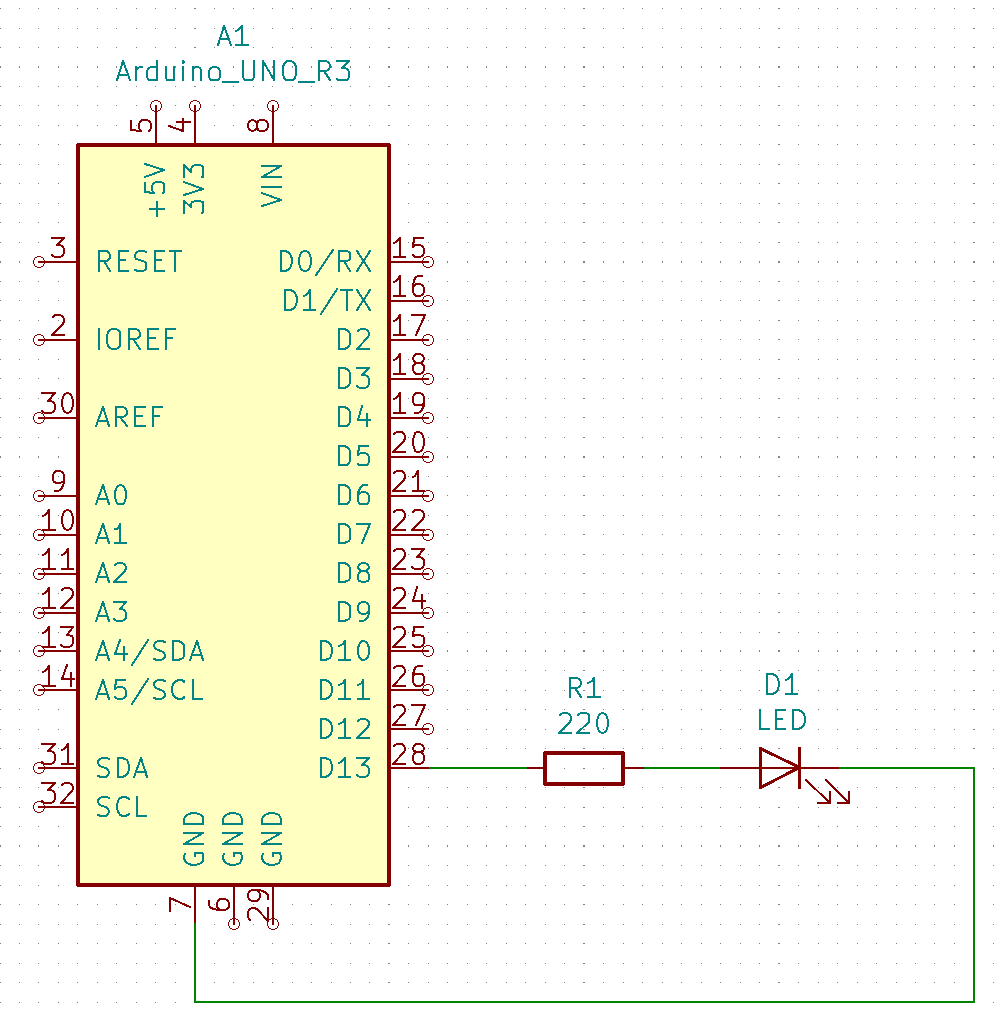https://cutelava.com/blogs/projects.atom Cute Lava - Projects 2019-07-02T16:24:00+08:00 Cute Lava https://cutelava.com/blogs/projects/how-to-fade-an-led-using-arduino 2019-07-02T16:24:00+08:00 2019-10-03T15:14:39+08:00 How to Fade an LED Using Arduino Amir Rajabifar In this example, we use the `analogWrite()` function to fade an LED on and off. `analogWrite()` uses pulse with modulation (PWM) to create an analog-like behavior from a digital output. This behavior is achieved by switching the output on and off very fast and with different ratio between on and off times.

More

]]>

In this example we use the `analogWrite()` function to fade an LED on and off. `analogWrite()` uses pulse with modulation (PWM) to create an analog-like behaviour from a digital output. This behaviour is achieved by switching the output on and off very fast and with different ratio between on and off times.  

## 1.0 Background Knowledge

### 1.1 Pulse With Modulation (PWM)

PWM is a technique that is used to generate analog signals with digital means. In this method a digital controller is used to create a square wave, a signal that switches between “on” and “off”. The generated on-off pattern simulates voltages between the two logic level, 5 Volts and 0 Volts. The pattern is generated by changing the portion of the time the signal is “on” versus the time that the signal is “off”. 

### 1.2 analogWrite()

Writes an analog value (PWM wave) to a pin. Can be used to light a LED at varying brightnesses or drive a motor at various speeds. After a call to `analogWrite()`, the pin will generate a steady square wave of the specified duty cycle until the next call to `analogWrite()` (or a call to `digitalRead()` or `digitalWrite()`) on the same pin. 

## 2.0 Hardware Required

• Arduino Board
• LED
• 220Ω Resistor
• Wires

## 3.0 Circuit

Connect the anode (the longer, positive leg) of your LED through a 220 \Omega resistor to digital output pin 11 on your board. Next, connect the cathode (the shorter, negative leg) of the LED directly to ground.## 4.0 Schematic## 5.0 Code

Declare pin 11 to be your `ledPin`.

the `analogWrite()` function requires two arguments: The first argument tells the function which pin it is writing to and the second argument indicates the PWM value.

In order to fade your LED on and off, you will need to gradually increase the PWM value from 0 (all the way off) to 255 (all the way on), and then back to 0 to complete the cycle.

`analogWrite()` can change the PWM value very fast. Thus, the delay at the end of the sketch controls the speed of fade. Try changing the amount of the delay and see how it changes the fading effect. 

## 6.0 References

"Arduino - Fading", Arduino.cc, 2019. [Online]. Available: https://www.arduino.cc/en/Tutorial/Fading. [Accessed: 28- Jun- 2019].

"Arduino - Fade", Arduino.cc, 2019. [Online]. Available: https://www.arduino.cc/en/Tutorial/Fade. [Accessed: 02- Jul- 2019].

"Arduino - PWM", Arduino.cc, 2019. [Online]. Available: https://www.arduino.cc/en/Tutorial/PWM. [Accessed: 02- Jul- 2019].

"Arduino Reference", Arduino.cc, 2019. [Online]. Available: https://www.arduino.cc/reference/en/language/functions/analog-io/analogwrite/. [Accessed: 02- Jul- 2019].

]]>
https://cutelava.com/blogs/projects/how-to-blink-an-led-using-arduino 2019-05-25T00:07:00+08:00 2020-01-30T14:37:10+08:00 How to Blink an LED Using Arduino Amir Rajabifar This project is the "Hello World!" of Arduino. In this project, we are going to learn about physical outputs and two electronic components, while performing the simplest project in the electronics sea of projects.

More

]]>
This project is the "Hello World!" of Arduino. In this project, we are going to learn about physical outputs and two electronic components, while performing the most straightforward project in the electronics sea of projects.

## 1.0 Hardware Required

• Arduino Board
• LED
• 220Ω Resistor

## 2.0 Background Knowledge

### 2.1 Series Circuits

In this project, components are connected in a series configuration. A series circuit provides only one path for current between two points so that the current is the same through each series component. 

### 2.2 Light Emitting diode - LED

A light-emitting diode (LED) is a semiconductor light source that emits light when current flows through it. 

### 2.3 Resistor

A resistor is a passive two-terminal electrical component that implements electrical resistance as a circuit element. In electronic circuits, resistors are used to reduce current flow, adjust signal levels, to divide voltages, bias active elements, and terminate transmission lines, among other uses. 

## 3.0 Circuit

To build the physical circuit for this project follow figure 1. Note that an Arduino Uno has been used in this circuit. However, any Arduino can be used.Figure 1 - Visual Circuit

1. Place resistor and LED on the breadboard.
2. Connect one leg of the resistor to digital pin 13 of the Arduino
3. Connect another leg of the resistor to the log leg of the LED (the positive leg, called anode)
4. Connect the short leg of the LED (the negative leg, called cathode) to the ground (called GND) 

## 4.0 Schematic## 5.0 Code

After you build the circuit plug your Arduino board into your computer, start the Arduino Software (IDE) and enter the code below. 

## 6.0 References

 T. L. Floyd, Principles of Electric Circuits, New Jersey: Pearson Education, Inc, 2010.

 "Light-emitting diode," wikipedia, [Online]. Available: https://en.wikipedia.org/wiki/Light-emitting_diode. [Accessed 20 05 2019].

 "Resistor," wikipedia, [Online]. Available: https://en.wikipedia.org/wiki/Resistor. [Accessed 20 05 2019].

 SM, "Blink," Arduino CC, 28 07 2015. [Online]. Available: https://www.arduino.cc/en/Tutorial/Blink. [Accessed 20 05 2019].

]]>